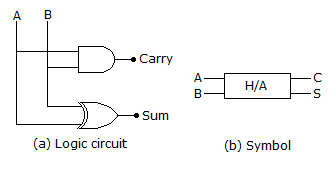# Electronics and Communication Engineering - Digital Electronics

41.

A digital system is required to amplify a binary encoded audio signal. The user should be able to control the gain of the amplifier from a minimum to a maximum in 100 increments. The minimum number of bits required to encode, in straight binary is,

 A. 8 B. 6 C. 5 D. 7

Answer: Option D

Explanation:

Let 6 bit required = 26 = 64, which indicate 64 increment.

If 7 bit = 27 = 128, for 100 increment, 7 bit required.

42.

A 4 bit transistor register has output voltage of high-low-high-low. The binary number stored and its decimal equivalent are

 A. 0101 and 5 B. 0101 and 10 C. 1010 and 5 D. 1010 and 10

Answer: Option D

Explanation:

1010 in binary and 8 + 2 = 10 in decimal.

43.

Assertion (A): A demultiplexer cannot be used as a decoder

Reason (R): A multiplexer selects one of many outputs whereas a decoder selects on output corresponding to coded input.

 A. Both A and R are correct and R is correct explanation of A B. Both A and R are correct but R is not correct explanation of A C. A is true, R is false D. A is false, R is true

Answer: Option D

Explanation:

Demux can be used as a decoder.

44.

Hexadecimal number F is called to octal number

 A. 15 B. 16 C. 17 D. 18

Answer: Option C

Explanation:

F = 15 in decimal notation = 17 in octal notation (8 + 7 = 15).

45.

A half adder can be used only for adding

 A. 1 s B. 2 s C. 4 s D. 8 s

Answer: Option A

Explanation:#### Current Affairs 2021

Interview Questions and Answers